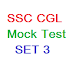SSC CGL mock test, free SSC CGL mock test, SSC CGL mock test online free, mock test for SSC CGL, SSC CGL mock test free online, free mock test for SSC CGL

Hi students, welcome to www.Coaching123.in ! In the previous class we have discussed SSC CGL Mock test SET#2. You can read the SET here-

In today's post we will discuss Mock test for SSC CGL SET#3. So, let's start the SET.

## Free SSC CGL mock test SET 3 - Maths

1. The ratio of the quantities of an acid and water in a mixture is 1 : 3. If 5 litres of acid is further added to the mixture, the new ratio becomes 1 : 2. The quantity of new mixture in litres is

a. 42

b. 45

c. 40

Ans. b

2. Monthly income of A and B are in the ratio of 4 : 3 and their expenses bear the ratio 3 : 2. Each of them saves Rs.6000 at the end of the month, then the monthly income of A is [very important question; same pattern asked in SSC CGL]

a. Rs.12000

b. Rs.24000

c. Rs.30000

Ans. b

3. The average of 18 observations is recorded as 124. Later it was found that two observations with values 64 and 28 were entered wrongly as 46 and 82. Find the correct average of the 18 observations. [very important question; same type pattern asked in SSC CGL]

a. 122

b. 123

c. 2196

Ans. a

4. If the cost price of 15 articles is equal to the selling price of 12 articles, find gain percentage

a. 20%

b. 25%

c. 18%

d. 21%

Ans. b

5. The cost price of an article is 64% of the marked price. The gain percentage after allowing a discount of 12% on the marked price is

a. 37.5%

b. 48%

c. 52%

d. 50.5%

Ans. a

6. Marked price of an article is Rs.275. Shopkeeper allows a discount of 5% and he gets a profit of 4.5%. The actual cost of the article is

a. 250

b. 225

c. 210

d. 215

Ans. a

7. A man purchased some eggs at 3 for Rs.5 and sold them at 5 for Rs.12. Thus he gained Rs.143 in all. The number of eggs he bought is

a. 210

b. 195

c. 200

d. 190

Ans. b

8. 20% loss in the selling price is what percent loss on the cost price?

a. 25%

b. 15%

c. 50 / 3 %

d. 49 / 3 %

Ans. c

9. A reduction in 20% in the price of sugar enables a man to purchase  5 kg more for Rs.600/-. Find the price of sugar per kg before reduction of price.

a. Rs.24

b. Rs.30

c. Rs.32

d. Rs.36

Ans. b

# Free SSC CGL Mock Test SET#3 | SSC CGL Coaching OnlineReviewed by ani on 6:53 AM Rating: 5